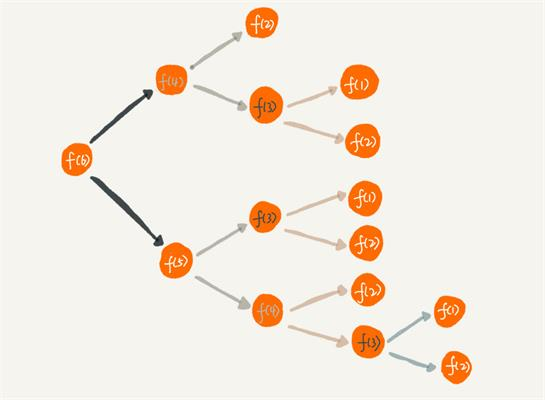# 关于递归的一些经验

## 递归的三大要素

### 第一要素：明确你这个函数想要干什么

// 算 n 的阶乘(假设n不为0)
int f(int n) {

}

### 第二要素：寻找递归结束条件

// 算 n 的阶乘(假设n不为0)
int f(int n) {
if(n == 1)
return 1;
}

// 算 n 的阶乘(假设n>=2)
int f(int n) {
if(n == 2)
return 2;
}

// 算 n 的阶乘(假设n不为0)
int f(int n) {
if(n <= 2)
return n;
}

### 第三要素：找出函数的等价关系式

// 算 n 的阶乘(假设n不为0)
int f(int n) {
if(n <= 2)
return n;

// 把 f(n) 的等价操作写进去
return f(n - 1) * n;
}

## 案例一：斐波那契数列

### 1、递归函数的功能

int f(int n) {

}

### 2、找出递归结束的条件

int f(int n) {
if(n <= 2)
return 1;
}

### 3、找出函数的等价关系式

int f(int n) {
// 1.先写递归结束条件
if(n <= 2)
return 1;

// 2.接着写等价关系式
return f(n - 1) + f(n - 2);
}

## 案例二：小青蛙跳台阶

### 1、递归函数的功能

int f(int n) {

}

### 2、找出递归结束的条件

int f(int n) {
if(n == 1)
return 1;
}

### 3、找出函数的等价关系式

int f(int n) {
if(n == 1) {
return 1;

return f(n - 1) + f(n - 2);
}

int f(int n) {
//f(0) = 0,f(1) = 1，等价于 n<=1时，f(n) = n。
if(n <= 1)
return n;

ruturn f(n - 1) + f(n - 2);
}

## 案例3：反转单链表。

class Node{
int data;
Node next;
}

### 1、递归函数的功能

Node reverseList(Node head) {

}

### 2. 寻找结束条件

Node reverseList(Node head) {
}

### 3. 寻找等价关系

Node reverseList(Node head) {

// 我们先把递归的结果保存起来，先不返回，因为我们还不清楚这样递归是对还是错
}

 //用递归的方法反转链表
public static Node reverseList2(Node head) {

// 递归反转 子链表
// 改变 1，2节点的指向
// 让 2 的 next 指向 1
// 1 的 next 指向 null
// 最后把反转之后的链表直接返回
return newList;
}

## 有关递归的一些优化思路

### 1. 考虑是否重复计算// 我们假定arr数组已经初始化好了
int f(int n) {
if(n <= 1)
return n;

//先判断有没计算过
if(arr[n] != -1)
//计算过，直接返回
return arr[n];
else {
// 没有计算过，递归计算,并且把结果保存到arr数组里
arr[n] = f(n - 1) + f(n - 1);
reutrn arr[n];
}
}

### 2. 考虑是否可以自底向上

public int f(int n) {
if(n <= 2)
return n;

int f1 = 1;
int f2 = 2;
int sum = 0;

for (int i = 3; i <= n; i++) {
sum = f1 + f2;
f1 = f2;
f2 = sum;
}
return sum;
}

## 最后总结

posted @ 2019-04-11 19:34  murphy_gb  阅读(...)  评论(...编辑  收藏